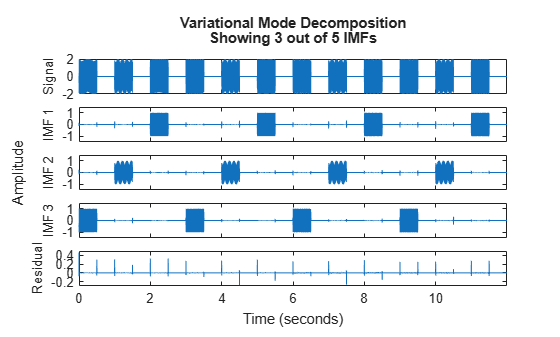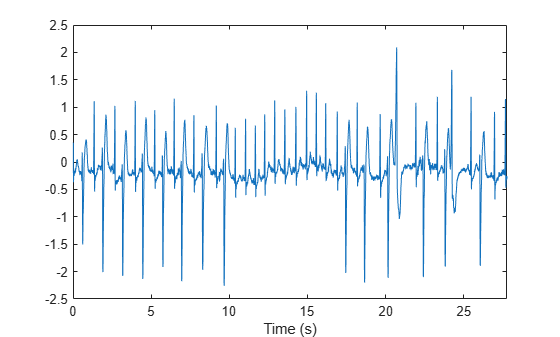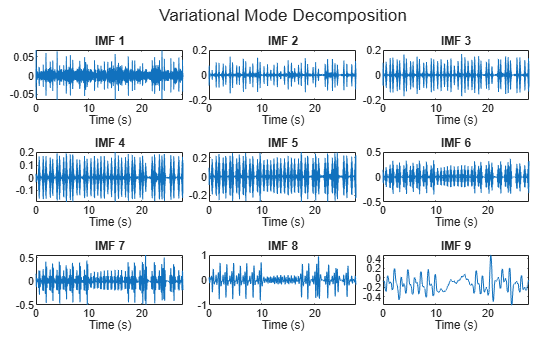# vmd

Variational mode decomposition

## Syntax

``imf = vmd(x)``
``[imf,residual] = vmd(x)``
``[imf,residual,info] = vmd(x)``
``[___] = vmd(x,Name,Value)``
``vmd(___)``

## Description

example

````imf = vmd(x)` returns the variational mode decomposition of `x`. Use `vmd` to decompose and simplify complicated signals into a finite number of intrinsic mode functions (IMFs) required to perform Hilbert spectral analysis.```
````[imf,residual] = vmd(x)` also returns the residual signal `residual` corresponding to the variational mode decomposition of `x`.```

example

````[imf,residual,info] = vmd(x)` returns additional information `info` on IMFs and the residual signal for diagnostic purposes.```

example

````[___] = vmd(x,Name,Value)` performs the variational mode decomposition with additional options specified by one or more `Name,Value` pair arguments.```

example

````vmd(___)` plots the original signal, IMFs, and the residual signal as subplots in the same figure.```

## Examples

collapse all

Create a signal, sampled at 4 kHz, that resembles dialing all the keys of a digital telephone. Save the signal as a MATLAB® timetable.

```fs = 4e3; t = 0:1/fs:0.5-1/fs; ver = [697 770 852 941]; hor = [1209 1336 1477]; tones = []; for k = 1:length(ver) for l = 1:length(hor) tone = sum(sin(2*pi*[ver(k);hor(l)].*t))'; tones = [tones;tone;zeros(size(tone))]; end end % To hear, type soundsc(tones,fs) S = timetable(tones,'SampleRate',fs);```

Plot the variational mode decomposition of the timetable.

`vmd(S)`Generate a multicomponent signal consisting of three sinusoids of frequencies 2 Hz, 10 Hz, and 30 Hz. The sinusoids are sampled at 1 kHz for 2 seconds. Embed the signal in white Gaussian noise of variance 0.01².

```fs = 1e3; t = 1:1/fs:2-1/fs; x = cos(2*pi*2*t) + 2*cos(2*pi*10*t) + 4*cos(2*pi*30*t) + 0.01*randn(1,length(t));```

Compute the IMFs of the noisy signal and visualize them in a 3-D plot.

```imf = vmd(x); [p,q] = ndgrid(t,1:size(imf,2)); plot3(p,q,imf) grid on xlabel('Time Values') ylabel('Mode Number') zlabel('Mode Amplitude')```Use the computed IMFs to plot the Hilbert spectrum of the multicomponent signal. Restrict the frequency range to [0, 40] Hz.

`hht(imf,fs,'FrequencyLimits',[0,40])`Generate a piecewise composite signal consisting of a quadratic trend, a chirp, and a cosine with a sharp transition between two constant frequencies at t = 0.5.

`$\mathit{x}\left(\mathit{t}\right)=6{\mathit{t}}^{2\text{\hspace{0.17em}}}+\mathrm{cos}\left(4\pi \mathit{t}+10\pi {\mathit{t}}^{2}\right)+\left\{\begin{array}{ll}\mathrm{cos}\left(60\pi \mathit{t}\right),& \mathit{t}\le 0.5,\\ \mathrm{cos}\left(100\pi \mathit{t}-10\pi \right),& \mathit{t}>0.5.\end{array}$`

The signal is sampled at 1 kHz for 1 second. Plot each individual component and the composite signal.

```fs = 1e3; t = 0:1/fs:1-1/fs; x = 6*t.^2 + cos(4*pi*t+10*pi*t.^2) + ... [cos(60*pi*(t(t<=0.5))) cos(100*pi*(t(t>0.5)-10*pi))]; tiledlayout('flow') nexttile plot(t,[zeros(1,length(t)/2) cos(100*pi*(t(length(t)/2+1:end))-10*pi)]) xlabel('Time (s)') ylabel('Cosine') nexttile plot(t,[cos(60*pi*(t(1:length(t)/2))) zeros(1,length(t)/2)]) xlabel('Time (s)') ylabel('Cosine') nexttile plot(t,cos(4*pi*t+10*pi*t.^2)) xlabel('Time (s)') ylabel('Chirp') nexttile plot(t,6*t.^2) xlabel('Time (s)') ylabel('Quadratic trend') nexttile(5,[1 2]) plot(t,x) xlabel('Time (s)') ylabel('Signal')```Perform variational mode decomposition to compute four intrinsic mode functions. The four distinct components of the signal are recovered.

```[imf,res] = vmd(x,'NumIMFs',4); tiledlayout('flow') for i = 1:4 nexttile plot(t,imf(:,i)) txt = ['IMF',num2str(i)]; ylabel(txt) xlabel('Time (s)') grid on end```

Reconstruct the signal by adding the mode functions and the residual. Plot and compare the original and reconstructed signals.

```sig = sum(imf,2) + res; nexttile(5,[1 2]) plot(t,sig,'LineWidth',1.5) hold on plot(t,x,':','LineWidth',2) xlabel('Time (s)') ylabel('Signal') hold off legend('Reconstructed signal','Original signal', ... 'Location','northwest')```Calculate the norm of the difference between the original and the reconstructed signals.

`norm(x-sig',Inf)`
```ans = 0 ```

The signals labeled in this example are from the MIT-BIH Arrhythmia Database . The signal in the database was sampled at 360 Hz.

Load the MIT database signals corresponding to record 200 and plot the signal.

```load mit200 Fs = 360; plot(tm,ecgsig) ylabel('Time (s)') xlabel('Signal')```The ECG signal contains spikes driven by the rhythm of the heartbeat and an oscillating low frequency pattern. The distinct spokes of the ECG create important higher order harmonics.

Calculate nine intrinsic mode functions of the windowed signal. Visualize the IMFs.

```[imf,residual] = vmd(ecgsig,'NumIMF',9); t = tiledlayout(3,3,'TileSpacing','compact','Padding','compact'); for n = 1:9 ax(n) = nexttile(t); plot(tm,imf(:,n)') xlim([tm(1) tm(end)]) txt = ['IMF',num2str(n)]; title(txt) xlabel('Time (s)') end title(t,'Variational Mode Decomposition')```The first mode contains the most noise, and the second mode oscillates at the frequency of the heartbeat. Construct a clean ECG signal by summing all but the first and last VMD modes, thus discarding the low frequency baseline oscillation and most of the high frequency noise.

```cleanECG = sum(imf(:,2:8),2); figure plot(tm,ecgsig,tm,cleanECG) legend('Original ECG','Clean ECG') ylabel('Time (s)') xlabel('Signal')```## Input Arguments

collapse all

Uniformly sampled time-domain signal, specified as either a vector or a timetable. If `x` is a timetable, then it must contain increasing finite row times.. The timetable must contain only one numeric data vector with finite load values.

Note

If a timetable has missing or duplicate time points, you can fix it using the tips in Clean Timetable with Missing, Duplicate, or Nonuniform Times.

### Name-Value Arguments

Specify optional comma-separated pairs of `Name,Value` arguments. `Name` is the argument name and `Value` is the corresponding value. `Name` must appear inside quotes. You can specify several name and value pair arguments in any order as `Name1,Value1,...,NameN,ValueN`.

Example: `'NumIMF',10`

Mode convergence absolute tolerance, specified as the comma-separated pair consisting of '`AbsoluteTolerance`' and a positive real scalar. `AbsoluteTolerance` is one of the stopping criteria for optimization, that is, optimization stops when the average squared absolute improvement toward convergence of IMFs, in two consecutive iterations, is less than `AbsoluteTolerance`.

Mode convergence relative tolerance, specified as the comma-separated pair consisting of '`RelativeTolerance`' and a positive real scalar. `RelativeTolerance` is one of the stopping criteria for optimization, that is, optimization stops when the average relative improvement toward convergence of IMFs, in two consecutive iterations, is less than `RelativeTolerance`.

Note

The optimization process stops when `'AbsoluteTolerance'` and `'RelativeTolerance'` are jointly satisfied.

Maximum number of optimization iterations, specified as the comma-separated pair consisting of '`MaxIterations`' and a positive scalar integer. `MaxIterations` is one of the stopping criteria for optimization, that is, optimization stops when the number of iterations is greater than `MaxIterations`.

`MaxIterations` can be specified using only positive whole numbers.

Number of IMFs extracted, specified as the comma-separated pair consisting of '`NumIMF`' and a positive scalar integer.

Initial central IMF frequencies, specified as the comma-separated pair consisting of '`CentralFrequencies`' and a vector of length `NumIMFs`. Vector values must be within [0, 0.5] cycles/sample, which indicates that the true frequencies are within [0, fs/2], where fs is the sample rate.

Initial IMFs, specified as the comma-separated pair consisting of '`InitialIMFs`' and a real matrix. The rows correspond to time samples and columns correspond to modes.

Penalty factor, specified as the comma-separated pair consisting of '`PenaltyFactor`' and a positive real scalar. This argument determines the reconstruction fidelity. Use smaller values of penalty factor to obtain stricter data fidelity.

Initial Lagrange multiplier, specified as the comma-separated pair consisting of '`InitialLM`' and a complex vector. The range of the initial Lagrange multiplier in the frequency domain is [0, 0.5] cycles/sample. The multiplier enforces the reconstruction constraint. The length of the multiplier depends on the input size.

Update rate for the Lagrange multiplier in each iteration, specified as the comma-separated pair consisting of '`LMUpdateRate`' and a positive real scalar. A higher rate results in faster convergence, but increases the chance of the optimization process getting stuck in a local optimum.

Method to initialize the central frequencies, specified as the comma-separated pair consisting of '`InitializeMethod`' and either `'peaks'`, `'random'`, or `'grid'`.

Specify `InitializeMethod` as:

• `'peaks'` to initialize the central frequencies as the peak locations of the signal in the frequency domain (default).

• `'random'` to initialize the central frequencies as random numbers distributed uniformly in the interval [`0,0.5`] cycles/sample.

• `'grid'` to initialize the central frequencies as a uniformly sampled grid in the interval [`0,0.5`] cycles/sample.

Toggle progress display in the command window, specified as the comma-separated pair consisting of '`Display`' and either `'true'` (or 1) or `'false'` (or 0). If you specify `'true'`, the function displays the average absolute and relative improvement of modes and central frequencies every 20 iterations, and show the final stopping information.

Specify `Display` as 1 to show the table or 0 to hide the table.

## Output Arguments

collapse all

Intrinsic mode functions, returned as a matrix or timetable. Each `imf` is an amplitude and frequency modulated signal with positive and slowly varying envelopes. Each mode has an instantaneous frequency that is nondecreasing, varies slowly, and is concentrated around a central value. Use `imf` to apply Hilbert-Huang transform to perform spectral analysis on the signal.

`imf` is returned as:

• A matrix where each column is an `imf`, when `x` is a vector

• A timetable, when `x` is a single data column timetable

Residual signal, returned as a column vector or a single data column timetable. `residual` represents the portion of the original signal `x` not decomposed by `vmd`.

`residual` is returned as:

• A column vector, when `x` is a vector.

• A single data column timetable, when `x` is a single data column timetable.

Additional information for diagnostics, returned as a structure with these fields:

• `ExitFlag` – Termination flag. A value of `0` indicates the algorithm stopped when it reached the maximum number of iterations. A value of `1` indicates the algorithm stopped when it met the absolute and relative tolerances.

• `CentralFrequencies` – Central frequencies of the IMFs.

• `NumIterations` – Total number of iterations.

• `AbsoluteImprovement` – Average squared absolute improvement toward convergence of the IMFs between the final two iterations.

• `RelativeImprovement` – Average relative improvement toward convergence of the IMFs between the final two iterations.

• `LagrangeMultiplier` – Frequency-domain Lagrange multiplier at the last iteration.

collapse all

### Intrinsic Mode Functions

The `vmd` function decomposes a signal x(t) into a small number K of narrowband intrinsic mode functions (IMFs):

`$x\left(t\right)=\sum _{k=1}^{K}{u}_{k}\left(t\right).$`

The IMFs have these characteristics:

1. Each mode uk is an amplitude and frequency modulated signal of the form

`${u}_{k}\left(t\right)={A}_{k}\left(t\right)\mathrm{cos}\left({\varphi }_{k}\left(t\right)\right),$`

where ϕk(t) is the phase of the mode and Ak(t) is its envelope.

2. The modes have positive and slowly varying envelopes.

3. Each mode has an instantaneous frequency ϕ'k(t) that is nondecreasing, varies slowly, and is concentrated around a central value fk.

The variational mode decomposition method simultaneously calculates all the mode waveforms and their central frequencies. The process consists of finding a set of uk(t) and fk(t) that minimize the constrained variational problem.

### Optimization

To calculate uk and fk, the procedure finds an optimum of the augmented Lagrangian

`$\begin{array}{ccccccc}L\left({u}_{k}\left(t\right),{f}_{k},\lambda \left(t\right)\right)& =& \alpha \sum _{k=1}^{K}{‖\frac{d}{dt}\left[\left(\delta \left(t\right)+\frac{j}{\pi t}\right)\ast {u}_{k}\left(t\right)\right]{e}^{-j2\pi {f}_{k}t}‖}_{2}^{2}& +& {‖x\left(t\right)-\sum _{k=1}^{K}{u}_{k}\left(t\right)\text{\hspace{0.17em}}‖}_{2}^{2}& +& 〈\lambda \left(t\right),x\left(t\right)-\sum _{k=1}^{K}{u}_{k}\left(t\right)〉\\ & & \left(i\right)& & \left(ii\right)& & \left(iii\right)\end{array},$`

where the inner product $〈p\left(t\right),q\left(t\right)〉={\int }_{-\infty }^{\infty }{p}^{\ast }\left(t\right)\text{\hspace{0.17em}}q\left(t\right)\text{\hspace{0.17em}}dt$ and the 2-norm ${‖p\left(t\right)‖}_{2}^{2}=〈p\left(t\right),p\left(t\right)〉$. The regularization term (i) includes these steps:

1. Use the Hilbert transform to calculate the analytic signal associated with each mode, where * denotes convolution. This results in each mode having a purely positive spectrum.

2. Demodulate the analytic signal to baseband by multiplying it with a complex exponential.

3. Estimate the bandwidth by calculating the squared 2-norm of the gradient of the demodulated analytic signal.

Terms (ii) and (iii) enforce the constraint $x\left(t\right)={\sum }_{k=1}^{K}{u}_{k}\left(t\right)$ by imposing a quadratic penalty and incorporating a Lagrange multiplier. The `PenaltyFactor` α measures the relative importance of (i) compared to (ii) and (iii).

The algorithm solves the optimization problem using the alternating direction method of multipliers described in .

## Algorithms

The `vmd` function calculates the IMFs in the frequency domain, reconstructing X(f) = DFT{x(t)} in terms of Uk(f) = DFT{uk(t)}. To remove edge effects, the algorithm extends the signal by mirroring half its length on either side.

The Lagrange multiplier introduced in Optimization has the Fourier transform Ʌ(f). The length of the Lagrange multiplier vector is the length of the extended signal.

Unless otherwise specified in `'InitialIMFs'`, the IMFs are initialized at zero. Initialize `'CentralFrequencies'` using one of the methods specified in `'InitializeMethod'`. `vmd` iteratively updates the modes until one of these conditions is met:

• $\sum _{k}{‖{u}_{k}^{n+1}\left(t\right)-{u}_{k}^{n}\left(t\right)‖}_{2}^{2}/{‖{u}_{k}^{n}\left(t\right)‖}_{2}^{2}<{\epsilon }_{\text{r}}$ and $\sum _{k}{‖{u}_{k}^{n+1}\left(t\right)-{u}_{k}^{n}\left(t\right)‖}_{2}^{2}<{\epsilon }_{\text{a}}$ are jointly satisfied, where εr and εa are specified using `'RelativeTolerance'` and `'AbsoluteTolerance'`, respectively.

• The algorithm exceeds the maximum number of iterations specified in `'MaxIterations'`.

For the (n + 1) -th iteration, the algorithm performs these steps:

1. Iterate over the K modes of the signal (specified using `'NumIMF'`) to compute:

1. The frequency-domain waveforms for each mode using

`${U}_{k}^{n+1}\left(f\right)=\frac{X\left(f\right)-\sum _{ik}{U}_{k}^{n}\left(f\right)+\frac{{\Lambda }^{n}}{2}\left(f\right)}{1+2\alpha {\left\{2\pi \left(f-{f}_{k}^{n}\right)\right\}}^{2}},$`

where ${U}_{k}^{n+1}\left(f\right)$ is the Fourier transform of the kth mode calculated in the (n + 1)-th iteration.

2. The kth central frequency ${f}_{k}^{n+1}$ using

`${f}_{k}^{n+1}=\frac{{\int }_{0}^{\infty }{|{U}_{k}^{n+1}\left(f\right)|}^{2}\text{\hspace{0.17em}}f\text{\hspace{0.17em}}df}{{\int }_{0}^{\infty }{|{U}_{k}^{n+1}\left(f\right)|}^{2}\text{\hspace{0.17em}}df}\approx \frac{\sum f{|{U}_{k}^{n+1}\left(f\right)|}^{2}\text{\hspace{0.17em}}}{\sum {|{U}_{k}^{n+1}\left(f\right)|}^{2}\text{\hspace{0.17em}}}.$`

2. Update the Lagrange multiplier using ${\Lambda }^{n+1}\left(f\right)={\Lambda }^{n}\left(f\right)+\tau \left(X\left(f\right)-\sum _{k}{U}_{k}^{n+1}\left(f\right)\right),$ where τ is the update rate of the Lagrange multiplier, specified using `'LMUpdateRate'`.

 Boyd, Stephen, Neal Parikh, Eric Chu, Borja Peleato, and Jonathan Eckstein. "Distributed Optimization and Statistical Learning via the Alternating Direction Method of Multipliers." Foundations and Trends® in Machine Learning. Vol 3, Number 1, 2011, pp. 1–122.

 Dragomiretskiy, Konstantin, and Dominique Zosso. "Variational Mode Decomposition." IEEE® Transactions on Signal Processing. Vol. 62, Number 3, 2014, pp. 531–534.

 Moody, George B., and Roger G. Mark. "The impact of the MIT-BIH Arrhythmia Database." IEEE Engineering in Medicine and Biology Magazine. Vol. 20, No. 3, May–June 2001, pp. 45–50.